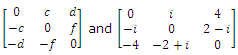# What is skew symmetric matrix

Publish On: 2019-03-17

Total Post: 559

# Mjay Jollay

Total Post: 0

## ANS: What is skew symmetric matrix

A square matrix A = [aij] is said to be symmetric or alternate matrix if A’ = - A.

i.e. aji = - aij for all values of i and j                       (1)

Putting j = i in (1), we get

aii = aii

i.e. 2aii = 0

or aii = 0 for all i.

This means that all the diagonal elements of a skew-symmetric matrix are zero.

The matricesare skew-symmetric matrices.